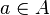# Blog Archives

## Surjection

A function is surjective (onto) if every possible image is mapped to by at least one argument. In other words, every element in the codomain has non-empty preimage. Equivalently, a function is surjective if its image is equal to its codomain. A surjective function is a surjection. The formal definition is the following.

The functionis surjective iff for all, there issuch that f(a) = b.
• A function f : AB is surjective if and only if it is right-invertible, that is, if and only if there is a function g: BA such that f o g = identity function on B. (This statement is equivalent to the axiom of choice.)
• By collapsing all arguments mapping to a given fixed image, every surjection induces a bijection defined on a quotient of its domain. More precisely, every surjection f : AB can be factored as a projection followed by a bijection as follows. Let A/~ be the equivalence classes of A under the following equivalence relation: x ~ y if and only if f(x) = f(y). Equivalently, A/~ is the set of all preimages under f. Let P(~) : AA/~ be the projection map which sends each x in A to its equivalence class [x]~, and let fP : A/~ → B be the well-defined function given by fP([x]~) = f(x). Then f = fP o P(~). A dual factorisation is given for injections above.
• The composition of two surjections is again a surjection, but if g o f is surjective, then it can only be concluded that g is surjective. See the figure at right*.

## A geometric proof of the impossibility of angle trisection by straightedge and compass

One of the most well known problems from ancient Greek mathematics was that of trisecting an angle by straightedge and compass, which was eventually proven impossible in 1837 by Pierre Wantzel, using methods from Galois theory.

Formally, one can set up the problem as follows. Define a configuration to be a finite collection${{\mathcal C}}$ of points, lines, and circles in the Euclidean plane. Define a construction step to be one of the following operations to enlarge the collection${{\mathcal C}}$:

• (Straightedge) Given two distinct points${A, B}$ in${{\mathcal C}}$, form the line${\overline{AB}}$ that connects${A}$ and${B}$, and add it to${{\mathcal C}}$.
• (Compass) Given two distinct points${A, B}$ in${{\mathcal C}}$, and given a third point${O}$ in${{\mathcal C}}$ (which may or may not equal${A}$ or${B}$), form the circle with centre${O}$ and radius equal to the length${|AB|}$ of the line segment joining${A}$ and${B}$, and add it to${{\mathcal C}}$.
• (Intersection) Given two distinct curves${\gamma, \gamma'}$ in${{\mathcal C}}$ (thus${\gamma}$ is either a line or a circle in${{\mathcal C}}$, and similarly for${\gamma'}$), select a point${P}$ that is common to both${\gamma}$ and${\gamma'}$ (there are at most two such points), and add it to${{\mathcal C}}$.

We say that a point, line, or circle is constructible by straightedge and compass from a configuration${{\mathcal C}}$ if it can be obtained from${{\mathcal C}}$ after applying a finite number of construction steps.

## SUBGROUP

In group theory, given a group G under a binary operation *, a subset H of G is called a subgroup of G if H also forms a group under the operation *. More precisely, H is a subgroup of G if the restriction of * to H x H is a group operation on H. This is usually represented notationally by HG, read as “H is a subgroup of G“.

A proper subgroup of a group G is a subgroup H which is a proper subset of G (i.e. HG). The trivial subgroup of any group is the subgroup {e} consisting of just the identity element. If H is a subgroup of G, then G is sometimes called an overgroup of H.

The same definitions apply more generally when G is an arbitrary semigroup, but this article will only deal with subgroups of groups. The group G is sometimes denoted by the ordered pair (G,*), usually to emphasize the operation * when G carries multiple algebraic or other structures.

In the following, we follow the usual convention of dropping * and writing the product a*b as simply ab.

## GROUP (MATHEMATICS)

In mathematics, a group is an algebraic structure consisting of a set together with an operation that combines any two of its elements to form a third element. To qualify as a group, the set and the operation must satisfy a few conditions called group axioms, namely closure, associativity, identity and invertibility. Many familiar mathematical structures such as number systems obey these axioms: for example, the integers endowed with the addition operation form a group. However, the abstract formalization of the group axioms, detached as it is from the concrete nature of any particular group and its operation, allows entities with highly diverse mathematical origins in abstract algebra and beyond to be handled in a flexible way, while retaining their essential structural aspects. The ubiquity of groups in numerous areas within and outside mathematics makes them a central organizing principle of contemporary mathematics. Read the rest of this entry